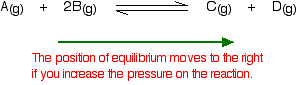## Monday, December 5, 2011

### What is a mole?

Today, we started talking about moles. Moles are used to count atoms. There are 22,000,000,000,000,000,000 quintillion atoms in a grain of sand and even counting grains of sand is a pain. Because atoms are so tiny, we use the mole to estimate.

There are 6.02 x 10 ^23 molecules in one mole. That's a whole lot. This is our new favorite number because it needs to be memorized. We practiced converting from moles to molecules.

Next we discussed molar mass. Molar mass = 1 mole and it also equals atomic mass from the periodic table. To find the molar mass of carbon dioxide you find the mass of carbon and two oxygens and add them together. Finding molar mass is not difficult unless the molecule has tricky subscripts (which we have been practicing).

## Friday, December 2, 2011

### Le Chatlier

Students learned about reaction rates and how to increase them. They also learned about reversible reactions and how Le Chatlier's principle influences shifts of equilibrium in reversible reactions.

Basically as you apply a stress to a system, the system will shift in response to the stress. If you add one of the molecules it will shift away from that molecule. If you take away a molecule, it will shift towards it to make more. Heat works the same way.Pressure is the tricky one. If pressure is applied to an equilibrium, then the reaction will shift to the side that has the least amount of molecules (count the coefficients).